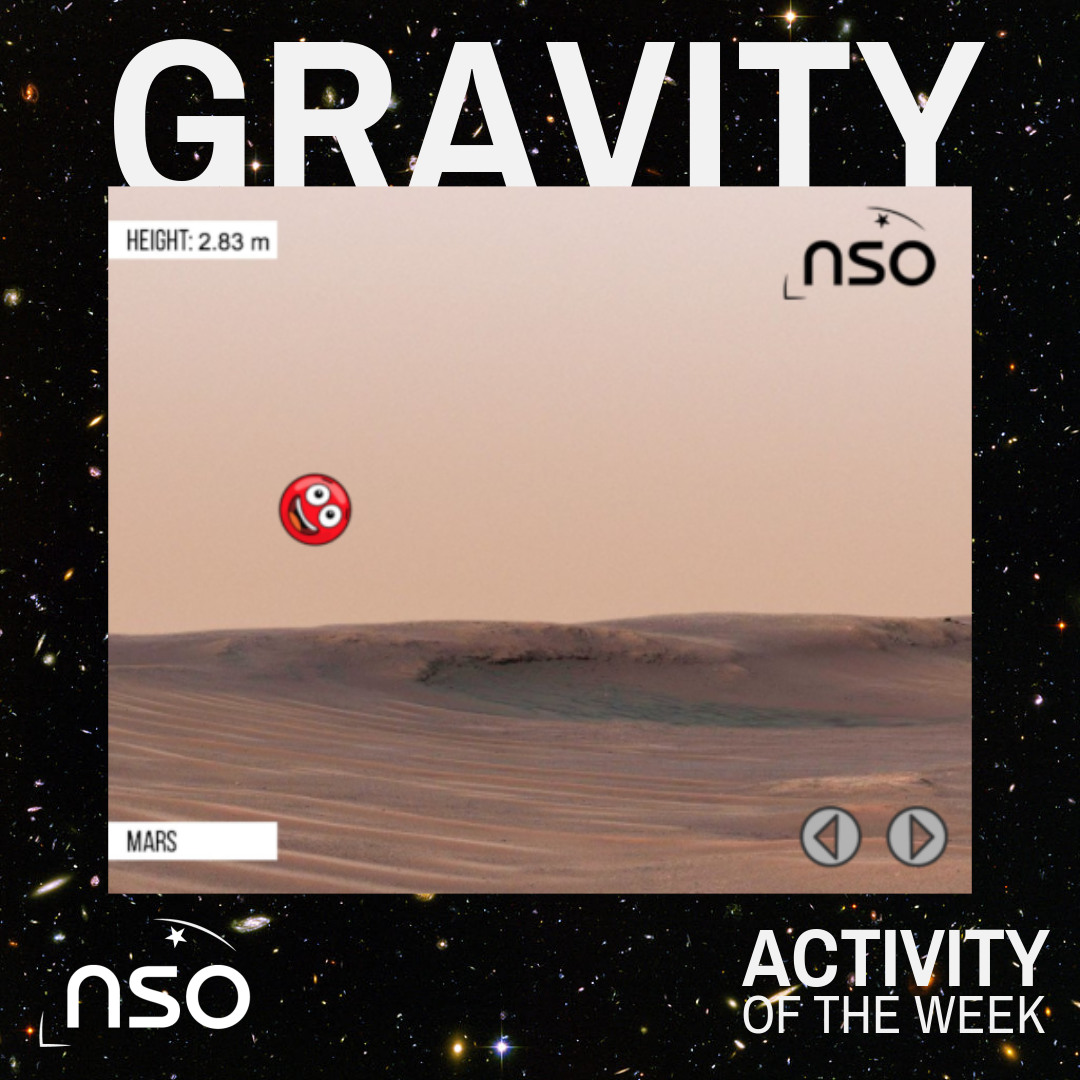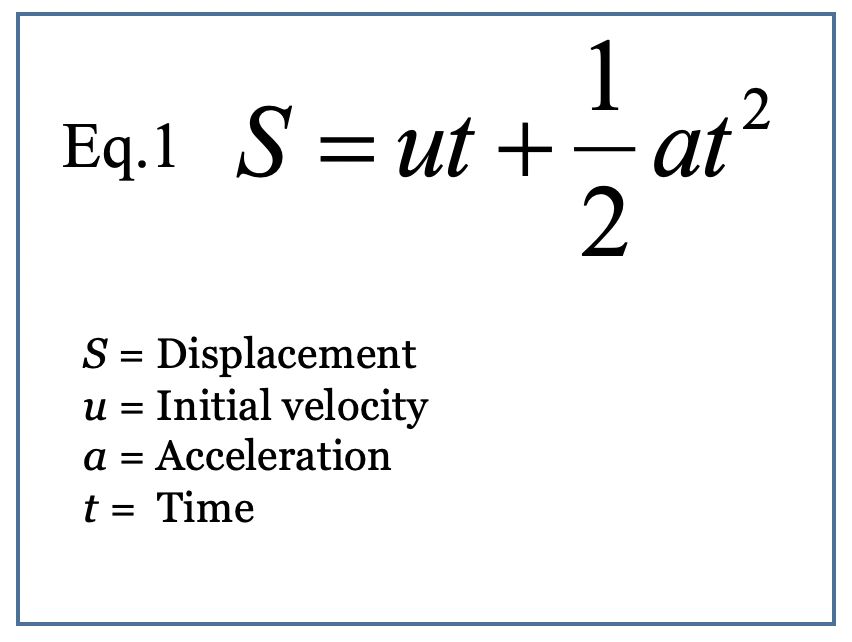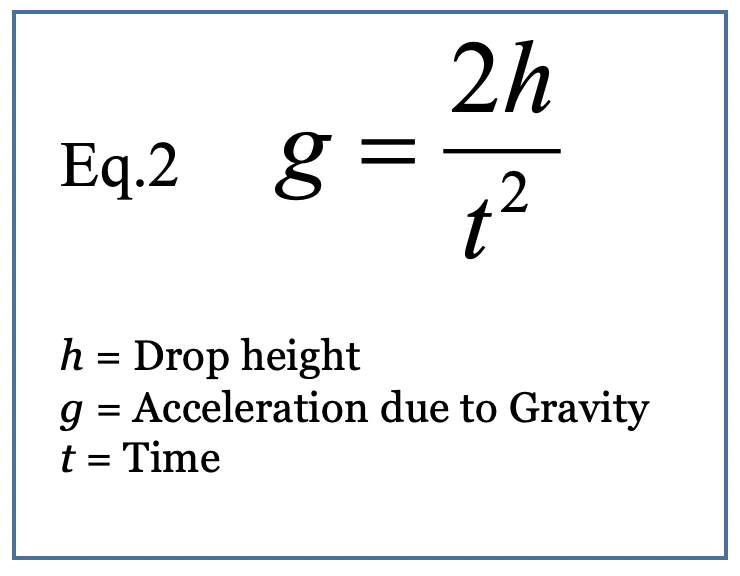#How does gravity make you feel on different planets?

Do you think you could bounce higher on Mercury than on Earth? Take a look at our Gravity Simulator and test it out for yourself.

The first part is really easy to do, go to our Gravity Simulator page, pick up the ball and drop it from the same spot on each planet/moon, time how long it takes to hit the bottom of the simulator (don't time any bounces) then try and answer these questions...

1. Where does the ball fall the fastest?
2. Where does the ball fall the slowest?
3. Where would be the most fun to use a trampoline?

Let us know on social media what you find out, you can tag us @SchoolsObs and use the hashtag #ShowTheNSO

Find out if your answers are correct by testing with an experiment. You will need the Gravity Simulator like you used above. Plus you will need a stopwatch and something to record your results on (you might find using a spreadsheet useful but pen and paper will do just fine too!).

The Experiment

1. In the Gravity Simulator, drop the ball from a set distance and measure the time it takes to hit the bottom of the box.
2. Repeat your measurements 3 times and record them.
3. Repeat for each of the planets in the simulator (and the Moon and Space).
4. Try testing how the ball reacts in each of the environments by throwing the ball with the mouse and note how this differs from Earth.
5. Analyse the results to obtain the value of the acceleration due to gravity for each planet (explanation below).

Analysing the Results

Values for the release height and the time taken to fall can be used to calculate the acceleration due to gravity for each of the planets. If it is assumed that there is no air resistance and no other downward force, the acceleration due to gravity is equal to a in Equation 1.

Since the ball has no initial velocity, u=0, the displacement, S, can be treated as the drop height, h.

Equation 1 can then be rearranged to allow calculation of the acceleration due to gravity giving Equation 2.Discussion Questions

In terms of gravity, which of the environments are most like Earth and which are the most different?

Why does gravity vary on other planets?

Was the gravity more difficult to measure on some planets than others?

What are the sources of error?